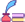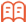##审稿期刊目次

## 期刊订阅

Open Access Article

Advances in International Applied Mathematics. 2022; 4: (2) ; 1-8 ; DOI: 10.12208/j.aam.20220008.

# Stochastic and convergence rate estimation at Zolotarev distance and its application to limit theoryZolotarev 距离下随机和收敛速率估计及其极限理论应用

*通讯作者： 贺钰淇,单位：中国人民大学统计学院 北京；四川大学数学学院 四川成都；

## Abstract

In recent years, stochastic sum and its limit theory have played a very important role in insurance, reliability theory and financial applications, so the research on the convergence rate of stochastic sum under the law of large numbers is also very critical. In this paper, a new metric Zolotarev distance is used to study the upper bound of the convergence rate of random sum distribution over Zolotarev distance. On this basis, the mixed Poisson sums proposed by Korolev and Zeifman are extended to the convergence rate of arbitrary random sums. Finally, the relationship between convergence under Zolotarev distance and convergence in distribution is discussed, and some applications of related random trees are discussed.

Key words： Zolotarev distance; random sum; convergence rate

## 参考文献 References

 Rényi, A. (1964). On an extremal property of the Poisson process. Annals of the Institute of Statistical Mathematics, 16(1), 129-133.

 Korolev, V. Y. (1996). Convergence of random sequences with independent random indices II. Theory of Probability Its Applications, 40(4), 770-772.

 Kalashnikov, V. V. (1997). Geometric sums: bounds for rare events with applications: risk analysis, reliability, queueing (Vol. 413). Springer Science Business Media.

 Brown, M. (1990). Error bounds for exponential approximations of geometric convolutions. The Annals of Probability, 1388-1402.

 Shevtsova, I., Tselishchev, M. (2020). A generalized equilibrium transform with application to error bounds in the Rényi theorem with no support constraints. Mathematics, 8(4), 577.

 苏淳, 冯群强, 刘杰, 2010. 现代极限理论及其在随机结构中的应用  . 高等 教育出版社.

 Wang D., Wu Z., 2006 . Moment complete convergence for sums of a sequence of NA random variables[J]. Applied Mathematics A Journal of Chinese Universities(Ser.A), 4(4):445-450.

 Dai Y., Guo M., 2017. Complete moment convergence for weighted sums of sequences of NOD random variables[J]. Journal of Anqing Normal University(Natural Science Edition), 023(002):36-39.

 Qiu D., Chen P., 2014. Complete moment convergence for i.i.d. random variables  . Statistics Probability Letters, 91:76-82.

 Bening, V. E. E., Korolev, V. Y. (2005). On an application of the Student distribution in the theory of probability and mathematical statistics. Theory of Probability Its Applications, 49(3), 377-391.

 Korolev, V., Shevtsova, I. (2012). An improvement of the Berry-Esseen inequality with applications to Poisson and mixed Poisson random sums. Scandinavian Actuarial Journal, 2012(2), 81-105.

 Schluter, C., Trede, M. (2016). Weak convergence to the Student and Laplace distributions. Journal of Applied Probability, 53(1), 121-129.

 Korolev, V., Zeifman, A. (2021). Bounds for convergence rate in laws of large numbers for mixed Poisson random sums. Statistics Probability Letters, 168, 108918.

 Aung, Y. Y., Min, M. M., 2018. Hybrid intrusion detection system using Kmeans and random tree algorithms. In 2018 19th IEEE/ACIS International Conference on Software Engineering, Artificial Intelligence, Networking and Parallel/Distributed Computing (SNPD) (pp. 218-223). IEEE.

 Probst, P., Wright, M. N., Boulesteix, A. L., 2019. Hyperparameters and tuning strategies for random forest. Wiley Interdisciplinary Reviews: data mining and knowledge discovery, 9(3), e1301.

 Heddam, S., Ptak, M., Zhu, S.,2020. Modelling of daily lake surface water temperature from air temperature: Extremely randomized trees (ERT) versus Air2Water, MARS, M5Tree, RF and MLPNN. Journal of Hydrology, 588, 125130.

 Cheng, H., Shi, Y., Wu, L., Guo, Y., Xiong, N., 2021. An intelligent scheme for big data recovery in Internet of Things based on multi-attribute assistance and extremely randomized trees. Information Sciences, 557, 66-83.

 Neininger, R., Rüschendorf, L. (2004). A general limit theorem for recursive algorithms and combinatorial structures. The Annals of Applied Probability, 14(1),